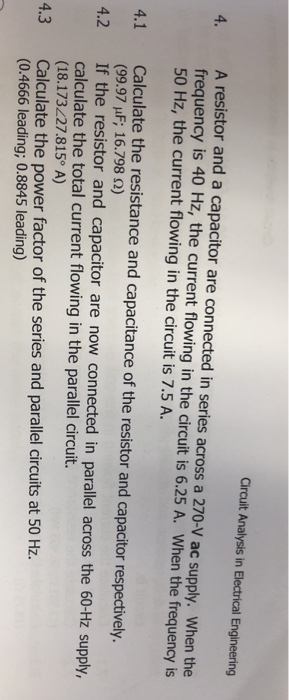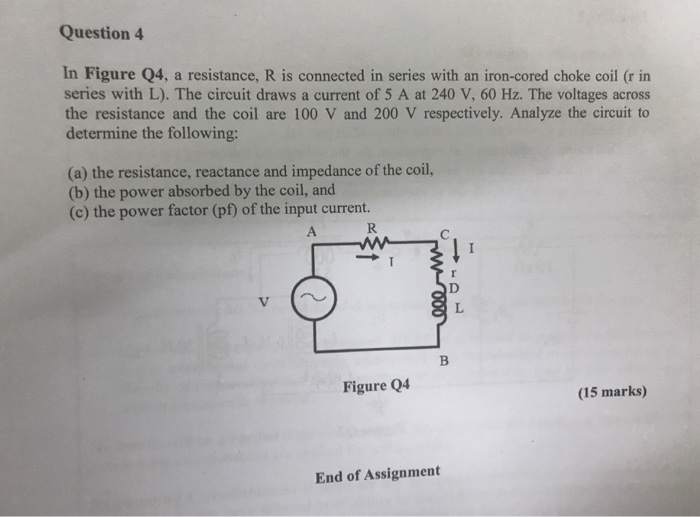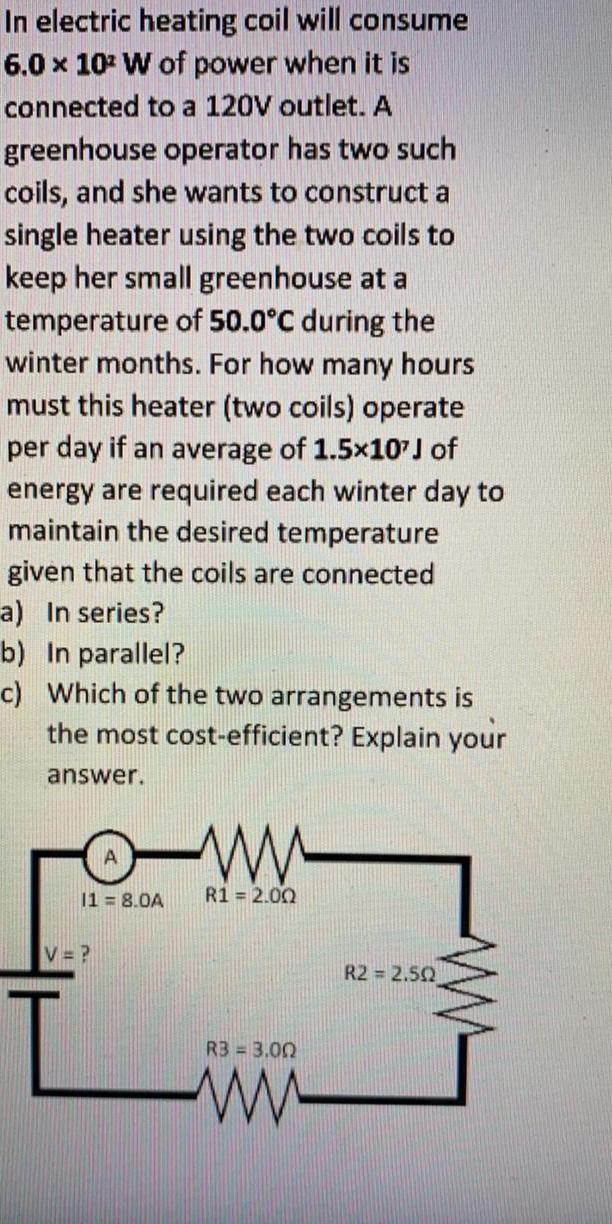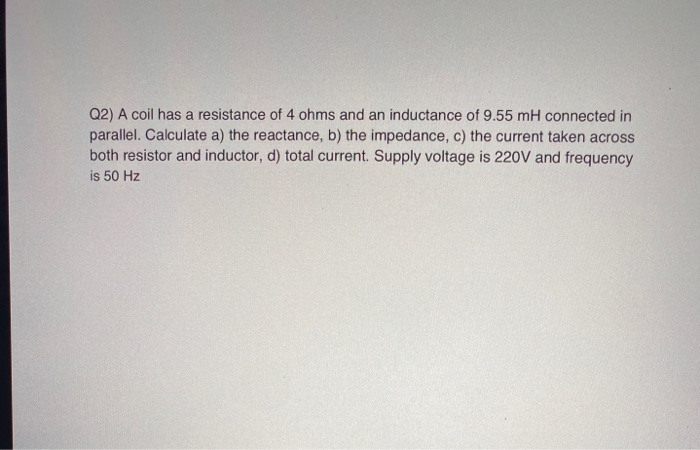Question

# A coil takes a current of 10.0 A and dissipates 1410 W when connected to a...

A coil takes a current of 10.0 A and dissipates 1410 W when connected to a 230 V, 50 Hz sinusoidal supply. When another coil is connected in parallel with it, the total current taken from the supply is 20.0 A at a power factor of 0.866. Determine the current and the overall power factor when the coils are connected in series across the same supply.

We need at least 10 more requests to produce the answer.

0 / 10 have requested this problem solution

The more requests, the faster the answer.

All students who have requested the answer will be notified once they are available.

#### Earn Coins

Coins can be redeemed for fabulous gifts.

Similar Homework Help Questions
• ### A coil takes a current of 10.0 A and dissipates 1410 W when connected to a...

A coil takes a current of 10.0 A and dissipates 1410 W when connected to a 230 V, 50 Hz sinusoidal supply. When another coil is connected in parallel with it, the total current taken from the supply is 20.0 A at a power factor of 0.866. Determine the current and the overall power factor when the coils are connected in series across the same supply.

• ### Two sinusoidal e.m.f.s of peak values 50 V and 20 V respectively but differing in phase...

Two sinusoidal e.m.f.s of peak values 50 V and 20 V respectively but differing in phase by 30° are induced in the same circuit. Draw the phasor diagram and ﬁnd the peak and r.m.s. values of the resultant e.m.f. A coil takes a current of 10.0 A and dissipates 1410 W when connected to a 230 V, 50 Hz sinusoidal supply. When another coil is connected in parallel with it, the total current taken from the supply is 20.0 A...

• ### Circuit Analysis in Electrical Engineering 4. A resistor and a capacitor are connected in series across a 270-V ac supply. When the frequency is 40 Hz, the current flowing in the circuit is 6.25...Circuit Analysis in Electrical Engineering 4. A resistor and a capacitor are connected in series across a 270-V ac supply. When the frequency is 40 Hz, the current flowing in the circuit is 6.25 A. When the frequency is 50 Hz, the current flowing in the circuit is 7.5 A Calculate the resistance and capacitance of the resistor and capacitor respectively. (99.97 μF; 16.798 Ω) If the resistor and capacitor are now connected in parallel across the 60-Hz supply, calculate...

• ### A heater dissipates 1200 W  of power when it is connected to a 190 V  battery. What current...

A heater dissipates 1200 W  of power when it is connected to a 190 V  battery. What current will flow through the heater when it is connected to the battery? What is R, the resistance of the heater? How long does it take to raise the temperature of the air in a good-sized living room that is 5.00 m  × 8.00 m ﻿ × 10.0 m ﻿ by 10.0∘C? Note that the specific heat of air is 1006 J/(kg⋅∘C) and the density of...

• ### Question 4 In Figure Q4, a resistance, R is connected in series with an iron-cored choke coil (r in series with L). The circuit draws a current of 5 A at 240 V, 60 Hz. The voltages across the res...Question 4 In Figure Q4, a resistance, R is connected in series with an iron-cored choke coil (r in series with L). The circuit draws a current of 5 A at 240 V, 60 Hz. The voltages across the resistance and the coil are 100 V and 200 V respectively. Analyze the circuit to determine the following (a) the resistance, reactance and impedance of the coil, (b) the power absorbed by the coil, and (c) the power factor (pf) of...

• ### In electric heating coil will consume 6.0 x 10- W of power when it is connected...In electric heating coil will consume 6.0 x 10- W of power when it is connected to a 120V outlet. A greenhouse operator has two such coils, and she wants to construct a single heater using the two coils to keep her small greenhouse at a temperature of 50.0°C during the winter months. For how many hours must this heater (two coils) operate per day if an average of 1.5x10') of energy are required each winter day to maintain the...

• ### a.When two resistors RA and RB are combined in series and connected to a battery it...

a.When two resistors RA and RB are combined in series and connected to a battery it is observed that the ratio of the power dissipated in RA to that dissipated in RB is equal to 10. If this arrangement is dismantled and the same two resistors connected in parallel to the same battery, what is the ratio of the power dissipated in RA to that dissipated in RB in this new circuit? b.How much energy is converted by a 12...

• ### A 250V, 100 Hz sinusoidal supply is connected to a parallel network comprising three branches A,...

A 250V, 100 Hz sinusoidal supply is connected to a parallel network comprising three branches A, B, and C as follows: A, a coil of resistance 10 ohms; B, a series circuit of 5 ohms and inductive reactance of 9 ohms; C, a series circuit of 6 ohms, inductance of 50mH, and a capacitive reactance of 10 ohms. Determine a) The apparent power of the combination b) The rating and value of the capacitor to be connected in parallel to...

Please help me to answer this question. TQ A coil having a resistance of 20Ω in series with an inductance of 0.0382H, is connected in parallel with a circuit consisting of a 150μF capacitor in series with a 10 Ω resistor. The arrangement is connected to a 240V, 50 Hz supply. Determine: The current in each branch. The total supply current. Draw the phasor diagram Calculate the apparent power, real power and reactive power of the circuit. Calculate the power...

• ### Q2) A coil has a resistance of 4 ohms and an inductance of 9.55 mh connected...Q2) A coil has a resistance of 4 ohms and an inductance of 9.55 mh connected in parallel. Calculate a) the reactance, b) the impedance, c) the current taken across both resistor and inductor, d) total current Supply voltage is 220V and frequency is 50 Hz